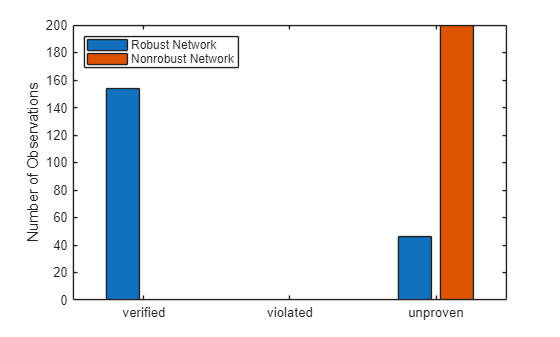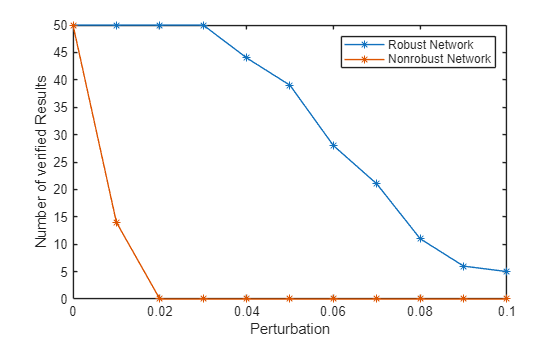# Verify Robustness of Deep Learning Neural Network

This example shows how to verify the adversarial robustness of a deep learning neural network.

Neural networks can be susceptible to a phenomenon known as adversarial examples , where very small changes to an input can cause the network predictions to significantly change. For example, making small changes to the pixels in an image can cause the image to be misclassified. These changes are often imperceptible to humans.

A network is adversarially robust if the output of the network does not change significantly when the input is perturbed. For classification tasks, adversarial robustness means that the output of the fully connected layer with the highest value does not change, and therefore the predicted class does not change .

In this example, you compare the robustness of a normal network and a network that is trained to be robust to adversarial examples.

This example requires the Deep Learning Toolbox™ Verification Library support package. If this support package is not installed, use the Add-On Explorer. To open the Add-On Explorer, go to the MATLAB® Toolstrip and click Add-Ons > Get Add-Ons.

Load a pretrained network. This network has been trained to classify images of digits.

`load("digitsClassificationConvolutionNet.mat")`

This network has a convolutional architecture with repeating sets of convolution, batch normalization and ReLU layers, followed by a global average pooling layer, and then a fully connected and softmax layer. The network is a `dlnetwork` object that has been trained using the first custom training loop from the Train Image Classification Network Robust to Adversarial Examples example with a learning rate of 0.1 and the max epochs set to 30.

Show the layers of the network.

`net.Layers`
```ans = 13×1 Layer array with layers: 1 'imageinput' Image Input 28×28×1 images 2 'conv_1' 2-D Convolution 10 3×3×1 convolutions with stride [2 2] and padding [0 0 0 0] 3 'batchnorm_1' Batch Normalization Batch normalization with 10 channels 4 'relu_1' ReLU ReLU 5 'conv_2' 2-D Convolution 20 3×3×10 convolutions with stride [2 2] and padding [0 0 0 0] 6 'batchnorm_2' Batch Normalization Batch normalization with 20 channels 7 'relu_2' ReLU ReLU 8 'conv_3' 2-D Convolution 40 3×3×20 convolutions with stride [2 2] and padding [0 0 0 0] 9 'batchnorm_3' Batch Normalization Batch normalization with 40 channels 10 'relu_3' ReLU ReLU 11 'gap' 2-D Global Average Pooling 2-D global average pooling 12 'fc' Fully Connected 10 fully connected layer 13 'softmax' Softmax softmax ```

You can use the `verifyNetworkRobustness` function to verify the adversarial robustness of the network. The function verifies the robustness with respect to the final layer. For most use cases, use the final fully connected layer for verification.

Prepare the network for verification by removing the softmax layer.

`net = removeLayers(net,"softmax");`

When you remove layers from a `dlnetwork` object, the software returns the network as an uninitialized `dlnetwork` object. To initialize the network, use the `initialize` function.

`net = initialize(net);`

Load test images of digits with which to verify the network.

`[XTest,TTest] = digitTest4DArrayData;`

Verification of the whole test set can take a long time. Use a subset of the test data for verification.

```numObservations = numel(TTest); numToVerify = 200; idx = randi(numObservations,numToVerify,1); X = XTest(:,:,:,idx); T = TTest(idx);```

Convert the test images to a `dlarray` object with the data format `"SSCB"` (spatial, spatial, channel, batch), which represents image data.

`X = dlarray(X,"SSCB");`

### Verify Network Robustness

To verify the adversarial robustness of a deep learning network, use the `verifyNetworkRobustness` function. The `verifyNetworkRobustness` function requires the Deep Learning Toolbox™ Verification Library support package.

To verify network robustness, the `verifyNetworkRobustness` function checks that, for all inputs between the specified input bounds, there does not exist an adversarial example. The absence of an adversarial example means that, for all images within the input set defined by the lower and upper input bounds, the predicted class label matches the specified label (usually the true class label).

For each set of input lower and upper bounds, the function returns one of these values:

• `"verified"` — The network is robust to adversarial inputs between the specified bounds.

• `"violated"` — The network is not robust to adversarial inputs between the specified bounds.

• `"unproven"` — The function cannot prove whether the network is robust to adversarial inputs between the specified bounds.

Create lower and upper bounds for each of the test images. Verify the network robustness to an input perturbation between –0.05 and 0.05 for each pixel.

```perturbation = 0.05; XLower = X - perturbation; XUpper = X + perturbation;```

Verify the network robustness for the specified input bounds and true class labels.

```result = verifyNetworkRobustness(net,XLower,XUpper,T); summary(result)```
``` verified 0 violated 0 unproven 200 ```
```figure bar(countcats(result)) xticklabels(categories(result)) ylabel("Number of Observations")```Adversarial training is a technique for training a network so that it is robust to adversarial examples . Load a pretrained network that has been trained to be robust to adversarial examples using the methods described in Train Image Classification Network Robust to Adversarial Examples. This network has the same layers as the normal network. The network has been trained to be robust to pixel perturbations in the range [–0.05, 0.05].

```load("digitsRobustClassificationConvolutionNet.mat") netRobust.Layers```
```ans = 13×1 Layer array with layers: 1 'imageinput' Image Input 28×28×1 images 2 'conv_1' 2-D Convolution 10 3×3×1 convolutions with stride [2 2] and padding [0 0 0 0] 3 'batchnorm_1' Batch Normalization Batch normalization with 10 channels 4 'relu_1' ReLU ReLU 5 'conv_2' 2-D Convolution 20 3×3×10 convolutions with stride [2 2] and padding [0 0 0 0] 6 'batchnorm_2' Batch Normalization Batch normalization with 20 channels 7 'relu_2' ReLU ReLU 8 'conv_3' 2-D Convolution 40 3×3×20 convolutions with stride [2 2] and padding [0 0 0 0] 9 'batchnorm_3' Batch Normalization Batch normalization with 40 channels 10 'relu_3' ReLU ReLU 11 'gap' 2-D Global Average Pooling 2-D global average pooling 12 'fc' Fully Connected 10 fully connected layer 13 'softmax' Softmax softmax ```

Prepare the network for verification using the same steps as for the normal network.

```netRobust = removeLayers(netRobust,"softmax"); netRobust = initialize(netRobust);```

Verify the network robustness.

```resultRobust = verifyNetworkRobustness(netRobust,XLower,XUpper,T); summary(resultRobust)```
``` verified 154 violated 0 unproven 46 ```

Compare the results from the two networks. The robust network has a greater number of observations that correspond to a `verified` result in comparison to the network without adversarial training.

```combineResults = [countcats(result),countcats(resultRobust)]; figure bar(combineResults) xticklabels(categories(result)) ylabel("Number of Observations") legend(["Normal Network","Robust Network"],Location="northwest")```### Compare Perturbation Values

Compare the number of verified results as the perturbation value changes. Create lower and upper bounds for each image for a range of perturbation values. To reduce computation time, specify multiple pairs of input bounds in a single call to the `verifyNetworkRobustness` function.

```numToVerify = 50; X = X(:,:,:,1:numToVerify); T = T(1:numToVerify); perturbationRange = 0:0.01:0.1; XLower = []; XUpper = []; TRange = []; j = 1; for i = 1:numel(perturbationRange) idxRange = j:(j+numToVerify-1); perturbationRangeIdx(i,1) = idxRange(1); perturbationRangeIdx(i,2) = idxRange(end); XLower(:,:,:,idxRange) = X - perturbationRange(i); XUpper(:,:,:,idxRange) = X + perturbationRange(i); TRange(idxRange) = T; j = j + numToVerify; end XLower = dlarray(XLower,"SSCB"); XUpper = dlarray(XUpper,"SSCB");```

Verify the robustness of both networks for each pair of input lower and upper bounds.

```result = verifyNetworkRobustness(net,XLower,XUpper,TRange); resultRobust = verifyNetworkRobustness(netRobust,XLower,XUpper,TRange);```

Find the number of verified results for each perturbation value.

```numVerified = []; numVerifiedRobust = []; for i = 1:numel(perturbationRange) range = perturbationRangeIdx(i,:); numVerified(i) = sum(result(range(1):range(2)) == "verified"); numVerifiedRobust(i) = sum(resultRobust(range(1):range(2)) == "verified"); end```

Plot the results. As the perturbation increases, the number of observations returning `verified` decreases for both networks.

```figure plot(perturbationRange,numVerified,"*-") hold on plot(perturbationRange,numVerifiedRobust,"*-") hold off legend(["Normal Network","Robust Network"]) xlabel("Perturbation") ylabel("Number of verified Results")```### References

 Goodfellow, Ian J., Jonathon Shlens, and Christian Szegedy. “Explaining and Harnessing Adversarial Examples.” Preprint, submitted March 20, 2015. https://arxiv.org/abs/1412.6572.

 Singh, Gagandeep, Timon Gehr, Markus Püschel, and Martin Vechev. “An Abstract Domain for Certifying Neural Networks.” Proceedings of the ACM on Programming Languages 3, no. POPL (January 2, 2019): 1–30. https://doi.org/10.1145/3290354.

 Madry, Aleksander, Aleksandar Makelov, Ludwig Schmidt, Dimitris Tsipras, and Adrian Vladu. “Towards Deep Learning Models Resistant to Adversarial Attacks.” Preprint, submitted September 4, 2019. https://arxiv.org/abs/1706.06083.